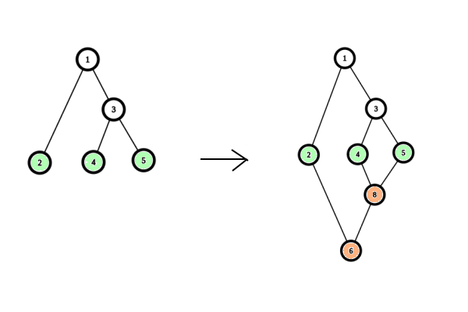# Meena Raju and Two Trees

Limits 2s, 512 MB

Meena's father gifted Meena and Raju two trees. Since they may quarrel, these two trees are exactly the same including label. Meena defines a node of a tree deaf node, if it has atmost one adjacent node.

Since these two trees are exactly the same, Meena used the following algorithm to connect these two trees and make a graph from them.

Let $n$ be the number of nodes in the tree. For every similar deaf node of the two trees, merge them into one node with the same label. By this way it creates one connected graph. But this graph may have some label of nodes twice. To solve this issue Raju suggested that, labels of Meena's tree remain exactly the same but for Raju's tree, the label of every node will be changed into previous label added by $n$. Note that, label of the deaf nodes will not change!

Now Meena and Raju have a graph with unique labels! Meena wants to find $q$ queries of the shortest path between node $a_i$ to $b_i$ ($1 \leq i \leq q$) in this graph.

For example, let's consider the tree of Meena and Raju as following:Then after merging these two trees, they will convert into the second graph.

## Input

First line will contain an integer T, ( $1 \leq T \leq 10$, the number of test cases).

For every test case, there will be two integers, $n \ q$ ($1 \leq n \leq 10^5$, the number of nodes in Meena and Raju's initial tree, $1 \leq q \leq 10^5$, the number of queries).

Following $n-1$ lines will contain two integers $u\ v$ (that means there is an edge between $u$ and $v$ in the tree).

The following $q$ lines each will contain $a_i\ b_i$ for $1 \leq i \leq q$ and $1 \leq a_i, b_i \leq 2n$, Meena's query nodes.

You can assume that all $a_i$ and $b_i$ are valid.

## Output

For each query, print a single line denoting the length of the shortest path between $a_i$ and $b_i$.

## Sample

InputOutput
2
5 2
1 2
1 3
3 4
3 5
3 8
2 5
3 2
1 2
1 3
1 4
2 4

2
3
2
1